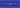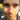# A Little Lazy Lambda Tutorial

April 22, 2017

javajava 8

Everyone is a bit lazy, so why not our code? Lambda expressions in Java 8 allow us to make our code that bit more lazy. In this short post I will cover how using Lambda expressions can make code more lazy.

If you have not used Lambda expressions before have a look at my some of my previous posts A Little Lambda Tutorial and Java 8 Streams for some background information not in this post.

So first things first, what does being lazy mean? We’ll I’m sure you know the answer as you have probably left things to the last minute and then quickly rushed to complete it at the end or even not do it at all. That is basically the definition of Lazy Evaluation, which defers the evaluation of a method until another expression requires it. If an expression never calls the lazy method then it will just lie around and do nothing. Java on the other hand uses Eager Evaluation by default and will execute a method as soon as it is called.

Lambdas allows Java to be lazy as they represent a function to be executed that can be passed around and will only be evaluated when required. The examples below are pretty simple but should demonstrate the order of execution showing that the Lambdas are not executed straight away.

If we start with a basic piece of code that is not using Lambdas we can see how they execute sequentially.

``````public class NonLazyCodeExample {
public static void main(String args[]) {

final int number = 4;
final boolean computeResult = compute(number);
final boolean processResult = process(number);

if (computeResult && processResult) {
System.out.println("TRUE");
} else {
System.out.println("FALSE");
}
}

public static boolean compute(final int number) {
System.out.println("computing number : " + number);
return number > 5;
}

public static boolean process(final int number) {
System.out.println("processing number : " + number);
return number % 3 == 0;
}
}``````

Which outputs

``````computing number : 4
processing number : 4
FALSE``````

This is due to both `compute` and `process` being called straight away when they are assigned to their variables. The execution of `process` was wasted as the second condition of the `if` statement was never reached. Obviously this example is extremely simple and most people will mention that the code can be rewritten to

``````public class NonLazyCodeExample {
public static void main(String args[]) {

final int number = 4;

if (compute(number) && process(number)) {
System.out.println("TRUE");
} else {
System.out.println("FALSE");
}
}

public static boolean compute(final int number) {
System.out.println("computing number : " + number);
return number > 5;
}

public static boolean process(final int number) {
System.out.println("processing number : " + number);
return number % 3 == 0;
}
}``````

Which will now output

``````computing number : 4
FALSE``````

As the `process` method is never called due to the `if` statement evaluating to `false` as soon as `compute` is executed. So why not do this in the first place? A possible reason against this is if longer methods are put into the `if` statement, maybe we a few parameters, it will start to get messy and you might struggle to read it. Whereas executing the methods earlier and assigning them to variables should make the `if` statement itself shorter and easier to understand.

Using Lambdas will combine both readability and removal of unneeded computations. By allowing us to define them to a variable without executing them. The example below should make this clearer.

``````public class LazyCodeExample {
public static void main(String args[]) {

final int number = 4;
final Supplier<Boolean> computeResult = () -> compute(number);
final Supplier<Boolean> processResult = () -> process(number);

if(computeResult.get() && processResult.get()) {
System.out.println("TRUE");
} else {
System.out.println("FALSE");
}
}

public static boolean compute(final int number) {
System.out.println("computing number : " + number);
return number > 5;
}

public static boolean process(final int number) {
System.out.println("processing number : " + number);
return number % 3 == 0;
}
}``````

Just for clarification the output is

``````computing number : 4
FALSE``````

As you can see this is the same output as the previous example showing that the same methods were executed in both. The `compute` and `process` methods are added to Lambda expressions which are stored in variables using the `Supplier` functional interface. The `Supplier` has a single method, `get`, which executes the Lambda that it represents.

Therefore when

``computeResult.get()``

Is called the function it represents is executed which in turn calls and executes the `compute` method. As this returned false the `if` statement also evaluated to false and `processResult.get` is never called leaving the `process` method to never be executed.

Another example can be shown with the use of a `Stream`which also will not be executed straight away.

``````public class LazyStreamExample {
public static void main(String args[]) {

final List<Integer> numbers = Arrays.asList(1, 2, 3, 4, 5, 6, 7, 8, 9);

System.out.println("DEFINING THE STREAM");

final Stream<Integer> stream =
numbers.stream()
.filter(LazyStreamExample::compute)
.filter(LazyStreamExample::process);

System.out.println("NOT EXECUTED YET");

final int result = stream.findFirst().orElse(0);

System.out.println("THE RESULT IS : " + result);
}

public static boolean compute(final int number) {
System.out.println("computing number : " + number);
return number > 5;
}

public static boolean process(final int number) {
System.out.println("processing number : " + number);
return number % 3 == 0;
}
}``````

``````DEFINING THE STREAM
NOT EXECUTED YET
computing number : 1
computing number : 2
computing number : 3
computing number : 4
computing number : 5
computing number : 6
processing number : 6
THE RESULT IS : 6``````

A `Stream` is created from the `numbers` list and stored within a variable. Like before with the `Supplier` it will not be executed until a dependent method is ran. In this example the `findFirst` method is used but unlike the `Supplier`, the `Stream` has many more methods that can execute it such as: `collect`, `findAny` and `count`.

Well done if you have made it to the end of this post (even thought it is short) and you have proved you are not lazy yourself… Or you scrolled to the end without actually reading it, in which case you are lazy. Anyway through the use of some lazy Lambdas we can keep our code easy to read without sacrificing performance by executing unneeded operations.Written by Dan Newton.